# macroeconomics

The table below shows the parameters for the economy of Hutu. Give your answers to one decimal point.

 C = 65 + 0.6Y XN = 190 − 0.1Y I = 155 G = 240

a. The value of equilibrium income is \$  .

b. If exports were to increase by 35, the new value of equilibrium income would be \$  .

c. Given your answer in part (b), the new value for XN is \$  .

d. Given the equilibrium income in part (a), if full employment income is \$1,000, what change in government spending is necessary to move the economy to this level?

Government spending needs to  by \$  .

We need at least 9 more requests to produce the answer.

1 / 10 have requested this problem solution

The more requests, the faster the answer.

All students who have requested the answer will be notified once they are available.

#### Earn Coin

Coins can be redeemed for fabulous gifts.

Similar Homework Help Questions
• ### The table below provides information for the economy of Zawi.

The table below provides information for the economy of Zawi. C = 25 + 0.6YXN = 15 − 0.1YI = 100G = 100a. The value of equilibrium income is \$ b. Set up a balancing row to verify your calculations (the tax equation is T = 80 + 0.15Y and X = 210). Enter your responses as whole numbers. YTYDCSIGXIMXNAE c. If exports decrease by 60, the new equilibrium income is \$

• ### KEYNESIAN MACROECONOMICS ( THE ROLE OF AGGREGATE DEMAND Derive and compare the mutipliers for two-secto...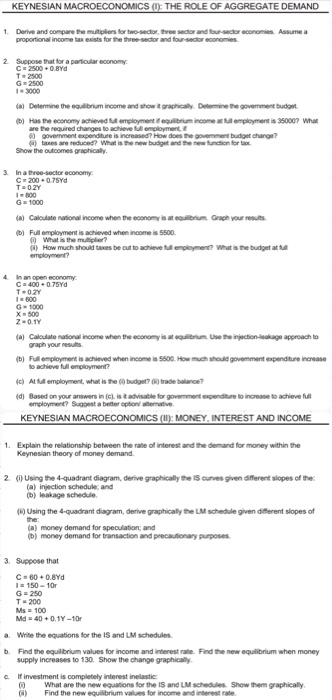KEYNESIAN MACROECONOMICS ( THE ROLE OF AGGREGATE DEMAND Derive and compare the mutipliers for two-sector, three sector and four-sector economies Assume a 2 Suppose that for a particular economy C 2500 T-2500 G 2500 3000 a) Determine the equlbrium income and show it graphicaly Detemine thegovemment budget b) Has the economy achieved ful employment equilibrium income a employment is 350007 Whr )taxes are reduced? What is the new budget and the new funcion forax Show the outcomes graphically C- 200...

• ### QUESTION 5 Which of the following can cause demand-pull inflation if the economy is currently in...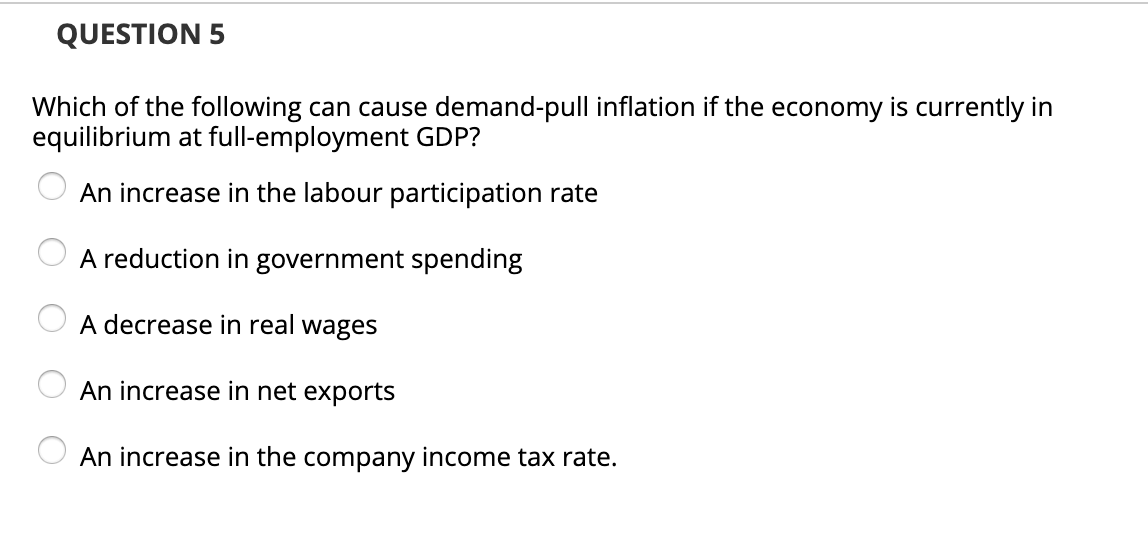QUESTION 5 Which of the following can cause demand-pull inflation if the economy is currently in equilibrium at full-employment GDP? O An increase in the labour participation rate O A reduction in government spending O A decrease in real wages O An increase in net exports O An increase in the company income tax rate.

• ### QUESTION 1 Suppose that the behavior of households, firms and the government in an economy is...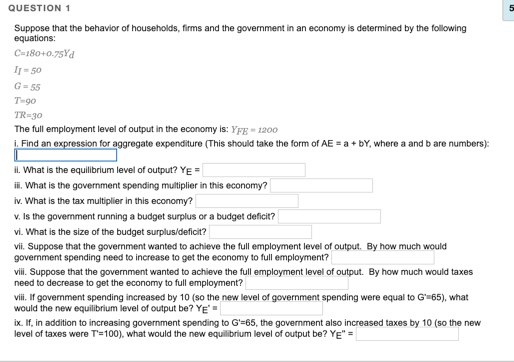QUESTION 1 Suppose that the behavior of households, firms and the government in an economy is determined by the following equations: C-180+0.75Y 1150 G-55 T-90 TWR30 The full employment level of output in the economy is: YFE 1200 Find an expression for aggregate expenditure (This should take the form of AE - abY, where a and b are numbers) il. What is the equilibrium level of output? YE = ill. What is the government spending multiplier in this economy? iv....

• ### (6) Imagine that the economy is in a recession. Which one of the following tactics is...

(6) Imagine that the economy is in a recession. Which one of the following tactics is a way to increase output by shifting aggregate demand outward? Raising taxes to increase the government surplus Increasing government spending Increasing the required reserve ratio Imposing tariffs on foreign goods          (7) In the short run, supply shocks cause prices to __________ and the quantity demanded to __________. increase; increase increase; decrease decrease; increase decrease; decrease          (8) Good deflation...

• ### f contractionary monetary policy is used, then which of the following would be most likely to...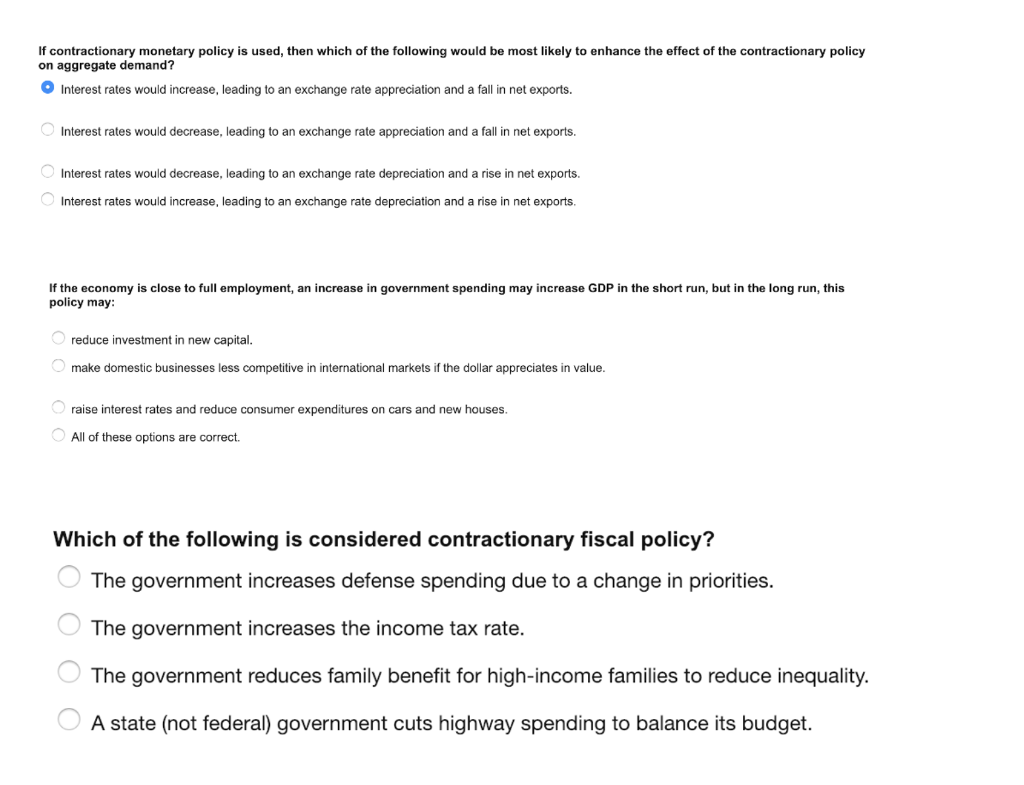f contractionary monetary policy is used, then which of the following would be most likely to enhance the effect of the contractionary policy on aggregate demand? Interest rates would increase, leading to an exchange rate appreciation and a fall in net exports. Interest rates would decrease, leading to an exchange rate appreciation and a fall in net exports. Interest rates would decrease, leading to an exchange rate depreciation and a rise in net exports. Interest rates would increase, leading to...

• ### The graph shows an economy below full employment. To restore full employment, the government incr...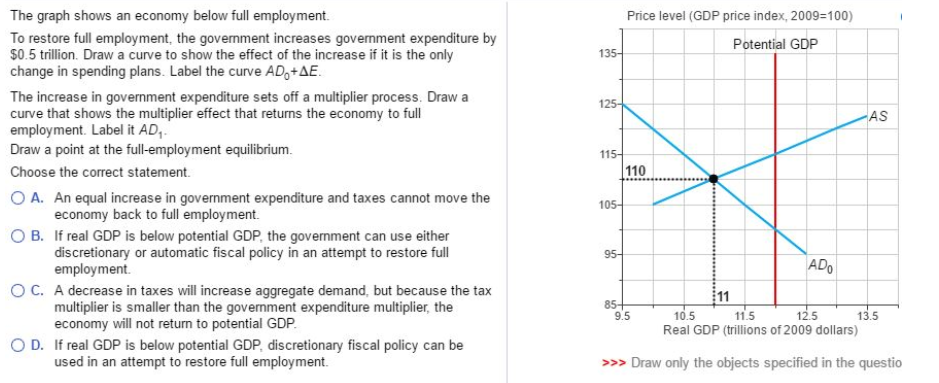The graph shows an economy below full employment. To restore full employment, the government increases government expenditure by \$0.5 trillion. Draw a curve to show the effect of the increase if it is the only change in spending plans. Label the curve ADo AE Price level (GDP price index, 2009-100) Potential GDP The increase in government expenditure sets off a multiplier process. Draw a curve that shows the multiplier effect that returns the economy to full employment. Label it AD,...

• ### Could you please help me with 1a-c. Pictures below. It would be greatly appreciated. Thankyou so...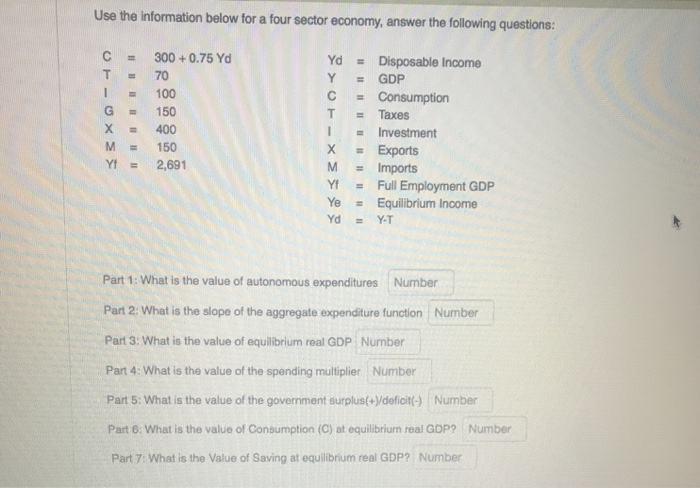Could you please help me with 1a-c. Pictures below. It would be greatly appreciated. Thankyou so much. 1.a) b) c) Use the information below for a four sector economy, answer the following questions: - 300 +0.75 Yd = = DELUXE 150 400 = Disposable Income GDP Consumption Taxes Investment Exports Imports Full Employment GDP Equilibrium Income 150 2,691 = YT Part 1: What is the value of autonomous expenditures Number Part 2: What is the slope of the aggregate expenditure...

• ### 1. To stimulate economic activity during a severe recession, the strongest appropriate fiscal policy is: a....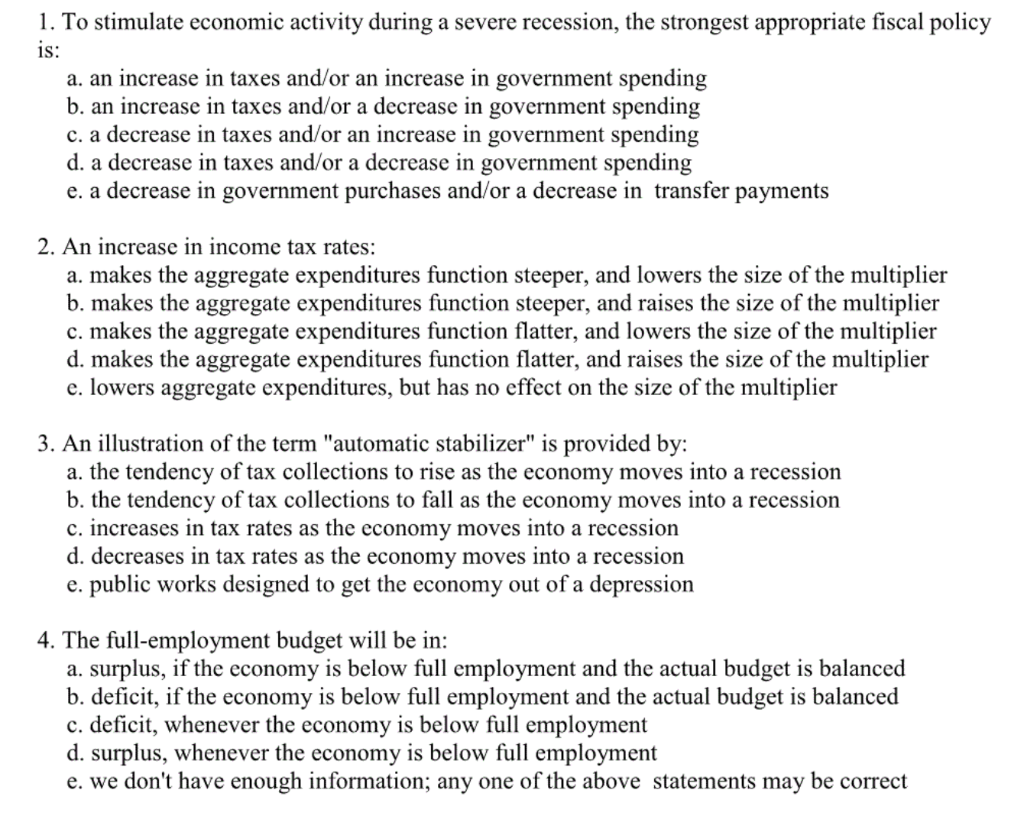1. To stimulate economic activity during a severe recession, the strongest appropriate fiscal policy is: a. an increase in taxes and/or an increase in government spending b. an increase in taxes and/or a decrease in government spending c. a decrease in taxes and/or an increase in government spending d. a decrease in taxes and/or a decrease in government spending e. a decrease in government purchases and/or a decrease in transfer payments 2. An increase in income tax rates: a. makes...

• ### 3. You are given the following information about the economy: autonomous consumption = \$300 billion      ...

3. You are given the following information about the economy: autonomous consumption = \$300 billion       planned investment = \$300 billion government spending = \$500 billion             mpc = .8 imports = \$200 billion                                    exports = \$500 billion                        a. Using the values above, what is the equation for the consumption function? b. Using the values above, what is the income/spending multiplier? c. What is the value of Net Exports? d. Is there a trade surplus or deficit? Of how much?...